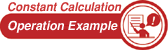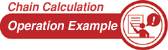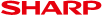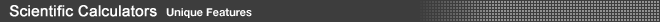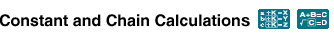Constant and Chain Calculation functions are popular in general electronic calculators as they allow equations to be entered without having to key repeatedly. Confusion may arise however, as not all scientific calculators show the equation being equal to the answer in the formula display. With SHARP's scientific calculators, the figures you omit are automatically shown as K (constant) or ANS (answer). Contradictions between equations and answers are eliminated and calculations are easier and more logical to enter.• Constant Calculation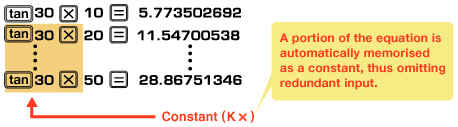• Chain Calculation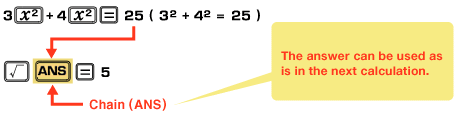(Example using EL-506T/X/W.)
 (N.B.) The EL-501X/W enables you to calculate the answer of the next equation just by pressing the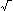key.